Courses

# Strength Of Material 2

## 20 Questions MCQ Test Mock Test Series for SSC JE Mechanical Engineering | Strength Of Material 2

Description
This mock test of Strength Of Material 2 for Mechanical Engineering helps you for every Mechanical Engineering entrance exam. This contains 20 Multiple Choice Questions for Mechanical Engineering Strength Of Material 2 (mcq) to study with solutions a complete question bank. The solved questions answers in this Strength Of Material 2 quiz give you a good mix of easy questions and tough questions. Mechanical Engineering students definitely take this Strength Of Material 2 exercise for a better result in the exam. You can find other Strength Of Material 2 extra questions, long questions & short questions for Mechanical Engineering on EduRev as well by searching above.
QUESTION: 1

### A rectangular bar of cross sectional area 10000 mm2 is subjected to an axial load of 40 kN. Determine the normal stress on the section which is inclined at an angle of 30° with normal cross-section of the bar.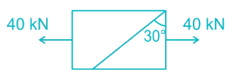Solution:

$$\sigma = \frac{P}{A} = \frac{{40 \times 1000}}{{1000}} = 4\;MPa$$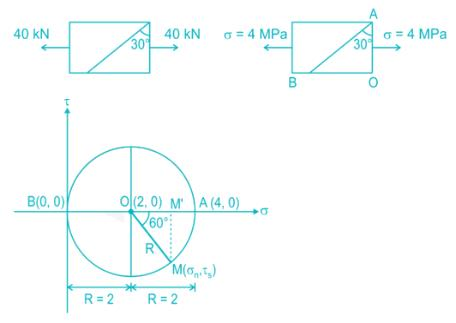Normal stress on inclined plane:

σn = BM' = R + R cos 60° = R(1 + cos 60)

σn = 2(1 + 0.5) = 3 MPa

Some more important calculations which may be asked in exams:

Shear stress on inclined plane:

$${\tau _s} = R\sin 60^\circ = \frac{{2 \times \sqrt 3 }}{2} = 1.732\;MPa$$

Maximum principal stress, σ1 = 4 MPa (θ = 0°)

Minimum principal stress, σ2 = 0 MPa (θ = 90°)

Radius of the Mohr circle R = σ1/2 = 2 MPa

Maximum shear stress, τmax = R = 2 MPa (θ = 45°)

QUESTION: 2

### For a thin spherical shell subjected to internal pressure, the ratio of volumetric strain to diametrical strain is _____.

Solution:

For thin cylinder: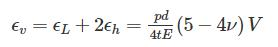For thin spherical shell: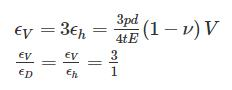QUESTION: 3

### For a rectangular section, the ratio of the maximum and average shear stresses is

Solution: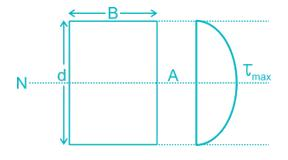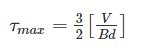τmax = 1.5 τavg

QUESTION: 4

Young’s modulus of elasticity is defined as the ratio of which of the following?

Solution:

Young's Modulus of Rigidity is the ratio of longitudinal stress to longitudinal strain.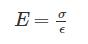Shear Modulus or Modulus of rigidity is the shear stress to shear strain.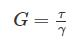Possion's ratio is the ratio of lateral strain to longitudinal stress.

Bulk Modulus of elasticity is the ratio of volumetric stress to volumetric strain.

QUESTION: 5

For beams of uniform strength, if depth is constant, then

Solution:

Using Flexural Formula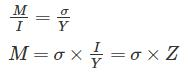M ∝ Z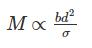d is constant

M ∝ b (width)

QUESTION: 6

According to maximum shear stress direction, at what ratio of maximum shear stress to yield stress of material does the yielding of material takes place?

Solution:

According to maximum shear stress criteria.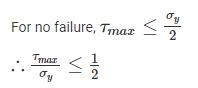QUESTION: 7

A cantilever beam is deflected by δ due to load P. If length of beam is doubled, the deflection compared to earlier case will be changed by a factor of:

Solution:

Deflection of cantilever beam having concentrated load at the free end is given by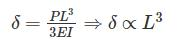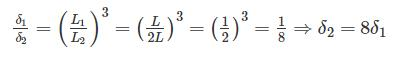QUESTION: 8

If a shaft turns at 150 rpm under a torque of 1500 Nm, then power transmitted is

Solution:Power=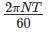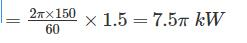QUESTION: 9

Use of strain rosette is to:

Solution:

Strain rosette can be defined as the arrangement strain gauge oriented at different angles. It only measures strain in one direction, to get principal strains, it is necessary to use a strain rosette.

Depending on the arrangement of strain gauges, strain rosettes are classified in to:-

1. Rectangular strain gauge rosette

2. Delta strain gauge rosette

3. Star strain gauge rosette

QUESTION: 10

A closed coil helical spring has 25 coils. If five coils of this springs are removed by cutting, the stiffness of the modified spring will

Solution: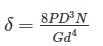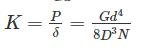K1N1 = K2N2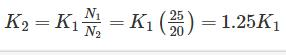QUESTION: 11

A tensile test is performed on a round bar. After fracture it has been found that the diameter remains approximately same at fracture. The material under test was

Solution:

In Brittle materials under tension test undergoes brittle fracture i.e there failure plane is 90° to the axis of load and there is no elongation in the rod that’s why the diameter remains same before and after the load. Example: Cast Iron, concrete etc

But in case of ductile materials, material first elongate and then fail, their failure plane is 45° to the axis of the load. After failure cup-cone failure is seen. Example Mild steel, high tensile steel etc.

QUESTION: 12

A rod of dimension 20 mm x 20 mm is carrying an axial tensile load of 10 kN. The tensile stress developed is ________.

Solution:

Stress=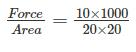=25N/mm2=25MPa\$

QUESTION: 13

A circular log of timber has diameter D. What will be the dimensions of the strongest rectangular section one can cut form this?

Solution: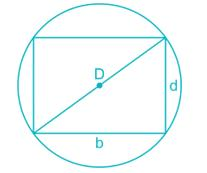For rectangular section: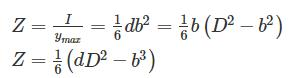The beam is strongest if section modulus is maximum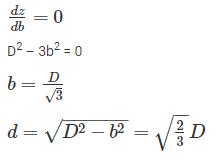Dimensions of strongest beam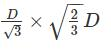QUESTION: 14

The correct shear force diagram for the cantilever beam with uniformly distributed load over the whole length of the beam is -

Solution: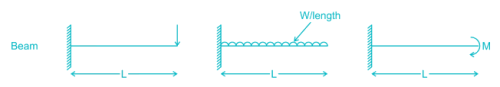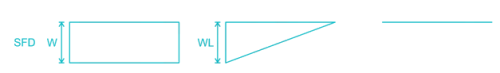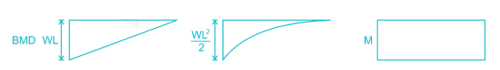QUESTION: 15

A cantilever beam 2 m in length is subjected to uniform load of 5 kN/m. If E = 200 GPa and I = 1000 cm, The strain energy stored is _____J

Solution: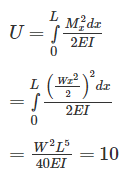QUESTION: 16

Effective length of a column effectively held in position and restrained in direction at one end but neither held in position nor restrained in direction at the other end is

Solution:

Given condition is “Effective length of a column effectively held in position and restrained in direction at one but neither held in position nor restrained in direction at the other end”

This implies one end fixed, other end freeleFF = 2L

QUESTION: 17

If the principle stresses on a plane stress problem are S1 = 100 MPa and S2 = 40 MPa then the magnitude of maximum shear stress (MPa) will be:

Solution:

In case of plane stress problem, Shear stress is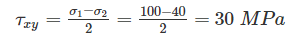QUESTION: 18

A spherical vessel with an inside diameter of 2 m is made of material having an allowable stress in tension of 500 kg/cm2. The thickness of a shell to with stand a pressure of 25 kg/cm2 should be:

Solution:

Allowable stress in spherical pressure vessel: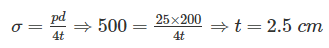QUESTION: 19

The bending moment diagram of a simply supported beam with a point load at centre is:

Solution: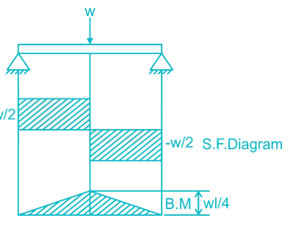QUESTION: 20

At a point in beam, the principle stresses are 100 N/mm2 and 50 N/mm2. The stress in x-direction is 65 N/mm2. The stress in y-direction will be

Solution:

In beam there is only stress in x and y direction, there is no stress in z direction.

σ1 + σ2 = σx + σy

100 + 50 = 65 + σy

σy = 150 – 65 = 85 N/mm2